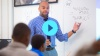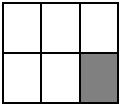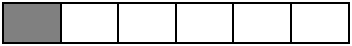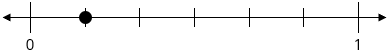# Fraction Equivalence and Ordering

Students are exposed to factors and multiples, then develop general methods and strategies to recognize and generate equivalent fractions, as well as learn to compare fractions with different numerators and different denominators.

Math

Unit 4

## Unit Summary

In this unit, students gain familiarity with factors and multiples, then use that understanding to develop general methods and strategies to recognize and generate equivalent fractions as well as to compare and order fractions.

Students began their study of fractions in Grades 1 and 2, where students learned to partition rectangles and circles into halves, thirds, and fourths. In Grade 3, students developed an understanding of fractions as numbers rather than simply equal parts of shapes. Students worked with number lines, which help to “reinforce the analogy between fractions and whole numbers” (Progressions for the Common Core State Standards in Math, p. 8). Students also began their work with recognizing and generating equivalent fractions in simple cases, using a visual fraction model to support that reasoning. This also involved the special case of whole numbers and various fractions, e.g., $${1=\tfrac{2}{2}=\tfrac{3}{3}=\tfrac{4}{4}...}$$.  Lastly, students began to compare fractions in cases where the two fractions have a common numerator or common denominator. Another key understanding from 3rd grade that students will rely on in this unit is their fluency with single-digit multiplication and division, aiding their understanding of factors and multiples and their relationship to fraction equivalence.

Students begin the unit by investigating factors and multiples within 100, as well as prime and composite numbers (4.OA.4). Then, in Topic B, students use their knowledge of factors and multiples as well as the fraction foundation built in Grade 3 to extend their understanding of and strategies to recognize and generate equivalent fractions. They use area models, tape diagrams, and number lines to understand and justify why two fractions $$\tfrac{a}{b}$$ and $$\tfrac{\left( n \ \times \ a \right)}{(n\ \times \ b)}$$ are equivalent, and they use those representations as well as multiplication and division to recognize and generate equivalent fractions. Lastly, they compare fractions with different numerators and different denominators in Topic C. They may do this by finding common numerators or common denominators. They may also compare fractions using benchmarks, such as “see[ing] that $$\tfrac{7}{8} < \tfrac{13}{12}$$ because $$\tfrac{7}{8}$$ is less than $$1$$ (and is therefore to the left of $$1$$) but $$\tfrac{13}{12}$$ is greater than $$1$$ (and is therefore to the right of $$1$$)” (Progressions for the Common Core State Standards in Math, p. 11). Throughout the discussion of fraction equivalence and ordering in Topics B and C, students’ work with factors and multiples, a supporting cluster content standard, engages them in the major work of fraction equivalence and ordering, e.g., by identifying a common factor of the numerator and denominator to generate an equivalent fraction in larger units.

Students engage with the practice standards in a variety of ways in this unit. For example, students construct viable arguments and critique the reasoning of others (MP.3) when they explain why a fraction $$\tfrac{a}{b}$$ is equivalent to a fraction $$\tfrac{\left( n \ \times \ a \right)}{(n\ \times \ b)}$$. Students use appropriate tools strategically (MP.5) when they choose from various models to solve problems. Lastly, students look for and make use of structure (MP.7) when considering how the number and sizes of parts of two equivalent fractions may differ even though the two fractions themselves are the same size.

Students will only work with fractions of the form $$\tfrac{a}{b}$$, including fractions greater than $$1$$. Students will develop an understanding of mixed numbers in Unit 5, where they will use fraction addition to see the equivalence of fractions greater than $$1$$ and mixed numbers. They also encounter all cases of addition and subtraction of fractions with like denominators, as well as multiplication of a whole number by a fraction in that unit. Then, in Unit 6, students will work with decimal fractions, developing an understanding of decimal notation for fractions, comparing decimal fractions, and adding decimal fractions with respective denominators $$10$$ and $$100$$. Students continue their work with fraction and decimal computation in Grades 5 and 6, including adding and subtracting fractions with unlike denominators by replacing the given fractions with equivalent ones. Thus, the property that "multiplying the numerator and denominator of a fraction by the same non-zero whole number results in a fraction that represents the same number as the original fraction" provides an important foundation for much of their upcoming work in Grade 4, as well as Grades 5 and 6 (Progressions for the Common Core State Standards in Math, p. 10).

Pacing: 17 instructional days (15 lessons, 1 flex day, 1 assessment day)

Fishtank Plus for Math

Unlock features to optimize your prep time, plan engaging lessons, and monitor student progress.## Assessment

The following assessments accompany Unit 4.

### Pre-Unit

Have students complete the Pre-Unit Assessment and Pre-Unit Student Self-Assessment before starting the unit. Use the Pre-Unit Assessment Analysis Guide to identify gaps in foundational understanding and map out a plan for learning acceleration throughout the unit.

### Mid-Unit

Have students complete the Mid-Unit Assessment after lesson 10.

### Post-Unit

Use the resources below to assess student mastery of the unit content and action plan for future units.

Expanded Assessment Package

Use student data to drive your planning with an expanded suite of unit assessments to help gauge students’ facility with foundational skills and concepts, as well as their progress with unit content.

## Unit Prep

### Intellectual Prep

Unit Launch

Prepare to teach this unit by immersing yourself in the standards, big ideas, and connections to prior and future content. Unit Launches include a series of short videos, targeted readings, and opportunities for action planning.#### Intellectual Prep for All Units

• Read and annotate “Unit Summary” and “Essential Understandings” portion of the unit plan.
• Do all the Target Tasks and annotate them with the “Unit Summary” and “Essential Understandings” in mind.
• Take the Post-Unit Assessment.

#### Unit-Specific Intellectual Prep

• When referred to fractions throughout Units 5 and 6, use unit language as opposed to “out of” language (e.g., $$\frac{3}{4}$$ should be described as “3 fourths” rather than “3 out of 4”). To understand why, read the blog post, Say What You Mean and Mean What You Say by Illustrative Mathematics.
• Read the blog post Fractions: Units and Equivalence from one of the lead writers of the Common Core State Standards, Bill McCallum.
• Read the following table that includes models used throughout the unit.
 Area model Example: The following shape represents 1 whole. $$\frac{1}{6}$$ of it is shaded.Tape diagram Example: The following shape represents 1 whole. $$\frac{1}{6}$$ of it is shaded.Number line Example: The point on the number line below is located at $$\frac{1}{6}$$.### Essential Understandings

• Every counting number is divisible by 1 and itself, and some counting numbers are also divisible by other numbers. Some counting numbers have exactly two factors (prime numbers); others have more than two (composite numbers). The numbers 0 and 1 are special cases in that they are neither prime nor composite. The product of any nonzero number and any other nonzero number is divisible by each number and is called a multiple of each number.
• “The numerical process of multiplying the numerator and denominator of a fraction by the same number, $$n$$, corresponds physically to partitioning each unit fraction piece into $$n$$ smaller equal pieces. The whole is then partitioned into $$n$$ times as many pieces, and there are $$n$$ times as many smaller unit fraction pieces as in the original fraction” (Progressions for the Common Core State Standards in Math, p. 6).
• “It is possible to over-emphasize the importance of simplifying fractions. There is no mathematical reason why fractions must be written in simplified form, although it may be convenient to do so in some cases” (Progressions for the Common Core State Standards in Math, p. 6).

### Vocabulary

benchmark fraction

common denominator

common numerator

composite number

factor pair

multiple

prime number

To see all the vocabulary for Unit 4, view our 4th Grade Vocabulary Glossary.

### Materials

• Random number generator (1 per student) — This can be a ten-sided die, a spinner of digits 0-9, Digit Cards for 0-9, or an online random number generator.
• Blank paper (1 sheet per student)
• Fraction Cards (1 per pair of students)

## Unit Practice

Word Problems and Fluency Activities

Access daily word problem practice and our content-aligned fluency activities created to help students strengthen their application and fluency skills.## Lesson Map

Topic A: Factors and Multiples

Topic B: Equivalent Fractions

Topic C: Comparing and Ordering Fractions

## Common Core Standards

Key

Major Cluster

Supporting Cluster

### Core Standards

#### Number and Operations—Fractions

• 4.NF.A — Extend understanding of fraction equivalence and ordering.
• 4.NF.A.1 — Explain why a fraction a/b is equivalent to a fraction (n × a)/(n × b) by using visual fraction models, with attention to how the number and size of the parts differ even though the two fractions themselves are the same size. Use this principle to recognize and generate equivalent fractions.
• 4.NF.A.2 — Compare two fractions with different numerators and different denominators, e.g., by creating common denominators or numerators, or by comparing to a benchmark fraction such as 1/2. Recognize that comparisons are valid only when the two fractions refer to the same whole. Record the results of comparisons with symbols >, =, or <, and justify the conclusions, e.g., by using a visual fraction model.

#### Operations and Algebraic Thinking

• 4.OA.B.4 — Find all factor pairs for a whole number in the range 1—100. Recognize that a whole number is a multiple of each of its factors. Determine whether a given whole number in the range 1—100 is a multiple of a given one-digit number. Determine whether a given whole number in the range 1—100 is prime or composite.

• 3.NF.A.1
• 3.NF.A.2
• 3.NF.A.3
• 4.NF.C.7

• 3.OA.C.7
• 4.OA.A.1

• 4.NF.B.3
• 4.NF.B.4
• 4.NF.C.5
• 4.NF.C.6
• 4.NF.C.7
• 5.NF.A.1
• 5.NF.B.5

### Standards for Mathematical Practice

• CCSS.MATH.PRACTICE.MP1 — Make sense of problems and persevere in solving them.

• CCSS.MATH.PRACTICE.MP2 — Reason abstractly and quantitatively.

• CCSS.MATH.PRACTICE.MP3 — Construct viable arguments and critique the reasoning of others.

• CCSS.MATH.PRACTICE.MP4 — Model with mathematics.

• CCSS.MATH.PRACTICE.MP5 — Use appropriate tools strategically.

• CCSS.MATH.PRACTICE.MP6 — Attend to precision.

• CCSS.MATH.PRACTICE.MP7 — Look for and make use of structure.

• CCSS.MATH.PRACTICE.MP8 — Look for and express regularity in repeated reasoning.

Unit 3

Multi-Digit Division

Unit 5

Fraction Operations